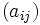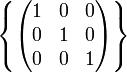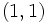# Subgroup structure of unitriangular matrix group:UT(3,p)

Jump to: navigation, search
This article gives specific information, namely, subgroup structure, about a family of groups, namely: unitriangular matrix group:UT(3,p).
View subgroup structure of group families | View other specific information about unitriangular matrix group:UT(3,p)

Let$p$ be an odd prime.

The group$G = U(3,p)$ is the group of unipotent upper-triangular matrices over the prime field of$p$ elements, and is also the unique (up to isomorphism) non-abelian group of order$p^3$ and exponent$p$. It is defined by the presentation:$G := \langle g,h,k \mid g^p = h^p = k^p = e, gh = hg, gk = kg, khk^{-1} = g^{-1}h \rangle$.

In the matrix description, each matrix$(a_{ij})$ can be described by the three entries$a_{12}, a_{13}, a_{23}$. The matrix looks like:$\begin{pmatrix} 1 & a_{12} & a_{13} \\ 0 & 1 & a_{23}\\ 0 & 0 & 1\end{pmatrix}$

The multiplication of matrices$A = (a_{ij})$ and$B = (b_{ij})$ gives the matrix$C = (c_{ij})$ where:

•$c_{12} = a_{12} + b_{12}$
•$c_{13} = a_{13} + b_{13} + a_{12}b_{23}$
•$c_{23} = a_{23} + b_{23}$

With the matrix description, we can set$g$ as the matrix with$a_{13} = 1$ and the other two entries zero,$h$ as the matrix with$a_{12} = 1$ and the other two entries zero, and$k$ as the matrix with$a_{23} = 1$ and the other two entries zero.

The group has the following subgroups:

## Tables for quick information

FACTS TO CHECK AGAINST FOR SUBGROUP STRUCTURE: (group of prime power order)
Lagrange's theorem (order of subgroup times index of subgroup equals order of whole group, so all subgroups have prime power orders)|order of quotient group divides order of group (and equals index of corresponding normal subgroup, so all quotients have prime power orders)
prime power order implies not centerless | prime power order implies nilpotent | prime power order implies center is normality-large
size of conjugacy class of subgroups divides index of center
congruence condition on number of subgroups of given prime power order: The total number of subgroups of any fixed prime power order is congruent to 1 mod the prime.

### Table classifying subgroups up to automorphisms

Automorphism class of subgroups Representative Isomorphism class Order of subgroups Index of subgroups Number of conjugacy classes Size of each conjugacy class Number of subgroups Isomorphism class of quotient (if exists) Subnormal depth (if subnormal)
trivial subgroup$\left \{ \begin{pmatrix} 1 & 0 & 0 \\ 0 & 1 & 0 \\ 0 & 0 & 1 \\\end{pmatrix}\right \}$ trivial group 1$p^3$ 1 1 1 prime-cube order group:U(3,p) 1
center of unitriangular matrix group:UT(3,p)$\langle \begin{pmatrix} 1 & 0 & 1 \\ 0 & 1 & 0 \\ 0 & 0 & 1 \\\end{pmatrix}\rangle$; equivalently, given by$a_{12} = a_{23} = 0$. group of prime order$p$$p^2$ 1 1 1 elementary abelian group of prime-square order 1
non-central subgroups of prime order in unitriangular matrix group:UT(3,p) Subgroup generated by any element with at least one of the entries$a_{12}, a_{23}$ nonzero group of prime order$p$$p^2$$p + 1$$p$$p(p + 1)$ -- 2
elementary abelian subgroups of prime-square order in unitriangular matrix group:UT(3,p) join of center and any non-central subgroup of prime order elementary abelian group of prime-square order$p^2$$p$$p + 1$ 1$p + 1$ group of prime order 1
whole group all elements unitriangular matrix group:UT(3,p)$p^3$ 1 1 1 1 trivial group 0
Total (5 rows) -- -- -- --$2p + 5$ --$p^2 + 2p + 4$ -- --

### Tables classifying isomorphism types of subgroups

Group name GAP ID Occurrences as subgroup Conjugacy classes of occurrence as subgroup Occurrences as normal subgroup Occurrences as characteristic subgroup
Trivial group$(1,1)$ 1 1 1 1
Group of prime order$(p,1)$$p^2 + p + 1$$p + 2$ 1 1
Elementary abelian group of prime-square order$(p^2,2)$$p + 1$$p + 1$$p + 1$ 0
Prime-cube order group:U3p$(p^3,3)$ 1 1 1 1
Total --$p^2 + 2p + 4$$2p + 5$$p + 4$$3$

### Table listing number of subgroups by order

Group order Occurrences as subgroup Conjugacy classes of occurrence as subgroup Occurrences as normal subgroup Occurrences as characteristic subgroup$1$ 1 1 1 1$p$$p^2 + p + 1$$p + 2$ 1 1$p^2$$p + 1$$p + 1$$p + 1$ 0$p^3$ 1 1 1 1
Total$p^2 + 2p + 4$$2p + 5$$p + 4$$3$